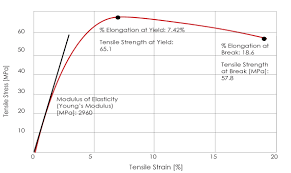## How to Calculate and Solve for Bulk Modulus of Elasticity (E) | Rock MechanicsThe image above represents bulk modulus of elasticity (E).

To compute for bulk modulus of elasticity (E), two essential parameters are needed and these parameters are young’s modulus (E) and Poisson’s ratio (v).

The formula for calculating the bulk modulus of elasticity (E):

k = E / 3(1 – 2v)

Where:

k = Bulk Modulus of Elasticity (E)
E = Young’s Modulus
v = Poisson’s Ratio

Let’s solve an example;
Find the bulk modulus of elasticity (E) when the young’s modulus is 66 and the Poisson’s ratio is 58.

This implies that;

E = Young’s Modulus = 66
v = Poisson’s Ratio = 58

k = E / 3(1 – 2v)
k = 66 / 3(1 – 2(58))
k = 66 / 3(1 – 116)
k = 66 / 3(-115)
k = 66 / -345
k = -0.19

Therefore, the bulk modulus of elasticity (E) is -0.19.

Calculating the Young’s Modulus when the Bulk Modulus of Elasticity (E) and the Poisson’s Ratio is Given.

E = k (3 – 6v)

Where:

E = Young’s Modulus
k = Bulk Modulus of Elasticity (E)
v = Poisson’s Ratio

Let’s solve an example;
Find the young’s modulus when the bulk modulus of elasticity (E) is 22 and the Poisson’s ratio is 18.

This implies that;

k = Bulk Modulus of Elasticity (E) = 22
v = Poisson’s Ratio = 18

E = k (3 – 6v)
E = 22 (3 – 6(18))
E = 22 (3 – 108)
E = 22 (-105)
E = -2376

Therefore, the young’s modulus is -2376.